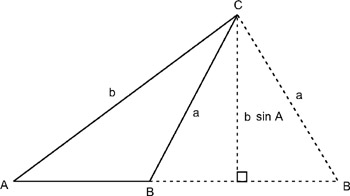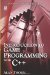# 3.10 Area of a Triangle

## 3.10 Area of a Triangle

The area of a triangle has been shown to be base × height divided by 2; or, rather, base × height multiplied by ½. This applies to all triangles. Consider the following triangle where the area must be considered in terms of the size and angles of a triangle. The height of this triangle can be considered to be an imaginary perpendicular drawn to the base.Figure 3.20

The perpendicular effectively divides the triangle into two right triangles, and the perpendicular represents the height. We therefore know the area of both right triangles, and the whole triangle is base × height × ½. The sine of angle θ is the ratio of the hypotenuse to the opposite side (the height). This ratio can therefore tell us the height:

• sin θ = h/a

Therefore:

• h = as in θ

Since h = a sin θ, this value can be substituted to find the area of a triangle, as:

 Area: A = ½bh Since: h = a sin θ Then: A = ½ba sin θ

Through experimenting with the above example you may notice that when perpendiculars are dropped at other angles on this triangle, it becomes possible to use the sine of any of the three angles in this triangle to calculate the area in this way. In short, the area of any triangle can be abbreviated to: half the product of two adjacent sides multiplied by the sine of their included angle. In other words, for the triangle with sides a, b, and c, and angles A, B, and C:

• Area = ½ ac sin B = ½ cb sin A = ½ ab sin CIntroduction to Game Programming with C++ (Wordware Game Developers Library)
ISBN: 1598220322
EAN: 2147483647
Year: 2007
Pages: 225
Authors: Alan Thorn

Similar book on Amazon

flylib.com © 2008-2017.
If you may any questions please contact us: flylib@qtcs.net